# Truth tables in PHP

All logical operators evaluate the truth value (that is, whether it is true or false) of each of the conditions separately, and then, according to the operator used to join these conditions, return a single value of true or false for the complete set of conditions that formed that expression.All logical operators evaluate the "truth value" (that is, whether it is true or false) of each of the conditions separately, and then, according to the operator used to join these conditions, return a single value of true or false for the complete set of conditions that formed that expression.

We are going to analyze four logical operators:

• And (conjunction, can also be written in PHP as &&),
• Or (disjunction, can also be written in PHP as ||),
• Xor (exclusive disjunction, informally called "excluding"),
• !(Denial).

If we give the symbolic name and the denominations \$ x and \$ y to the two conditions that we will join using logical operators, the truth values can only be four:

• That both conditions are true,
• That both conditions are false,
• That the first is true and the second false,
• That the first is false and the second true. There is no other alternative. This can be graphed with this table: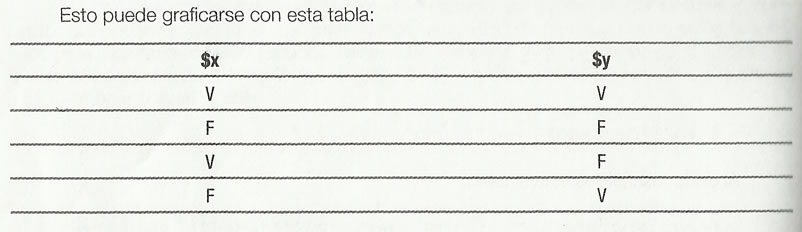(Being "V" true, and "F" false). Depending on which operator we use to join both conditions, we will obtain different results when evaluating the complete set. Let's see what is the "truth table" to join conditions, according to each of these logical operators.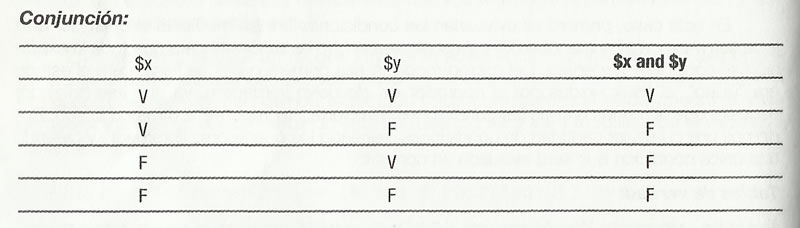The conjunction operator is a fairly restrictive logical operator, since it requires that all the conditions that are part of the expression be true so that the complete condition returns true.

In any other case, that is, with only one of the conditions being false, the complete expression is evaluated as false.

Let's see an example with PHP. Suppose we have these data:

\$user="pepe";
\$key=123456;

And we want to validate them with a conditional that evaluated if the name is "pepe" and the key is "123456". We need both of them to be true to give the whole condition as true and give access to that user, so we will use a conjunction operator:

if(\$name=="pepe" and \$key=="123456") {
print("¡Welcome Pepe!");
} else {
print("We do not know you, we’re sorry but you’re out");
}

It is recommended that we test what happens when we provide "false" values (different from those expected in the condition). For example, we could change the values of those two variables in this way:

\$user="Juan";
\$key=123456;

In that case, the condition would be evaluated as false, since the first condition (that \$ name is "pepe") is evaluated as false, so, even if the second is true, having joined both with a conjunction operator and, the entire condition is evaluated as false.

In the same way, we could change the values of those two variables again, this time to something like.

\$user="Pepe";
\$key=99999;

And also, in this case, the condition would be evaluated as false, since the first condition is true, but the second is false.

Of course, it would also be assessed as false if both parties were false (both the name and the key).The disjunction operator, contrary to the previous one, is a fairly broad logical operator, since it considers that the expression is true if at least one of the conditions that is part of the expression is true.

The only way for a disjunction to be false is that all conditions are false, without exception.

Let's see an example with PHP, imagine that an e-commerce site makes home deliveries only in three cities: city 1, city 2 and city 3; We ask the user to enter their city of residence in a field called "city", and evaluate that data. We need at least one of those three city names to be the one that actually entered the user to take the entire condition as true, and place the order. Then, we will use a disjunction operator:

if(\$_POST["city"]=="city1" or \$_POST["city"]=="city2" or \$_POST["city"]=="city3"){

print("Correct area! You will receive your order.");
} else {
print("This is outside the coverage area.");

}

Again, it is recommended that we try changing the expected values, and that we see what happens when we provide false values or one of the true ones. In the case of a disjunction, with which we will provide only one of the correct values, the expression will be evaluated as correct.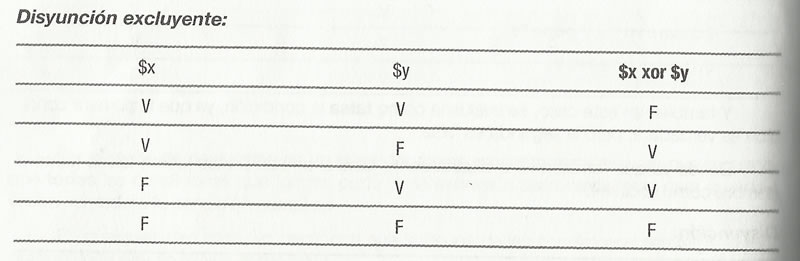The difference with the operator of the common disjunction is that if both conditions are true, it returns false.

Suppose we have this information provided in an order:

\$card="VISA";
\$coupon="19876";

And we want to validate them with the rule that if you pay with a VISA card, no discount coupon is considered. On the contrary, if you enter a discount coupon, the form of payment can not be VISA. They are exclusive:

if (\$card == "VISA" xor \$coupon <> ""){
print("We can take your order");
} else {
print("You cannot choose VISA and at the same time place a discount coupon code, and you cannot choose another means of payment without entering a coupon code.");
}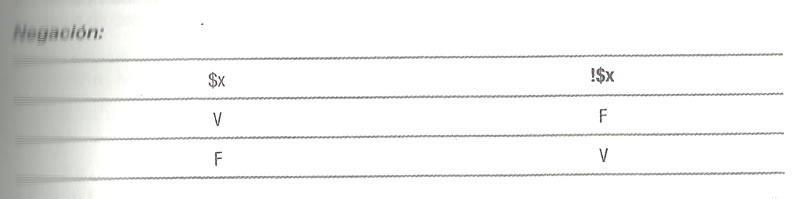The operator of negation transforms the expression into its opposite. That is, if the condition is true, it returns false, and if the condition is false, it returns true.

We have already used this operator in other cases, such as:

if (\$_POST["nombre"] != "Pepe" {
print ("No sos Pepe");

}

Returns true if name is not equal to "Pepe".
Let us summarize, then, in a table, the different logical operators: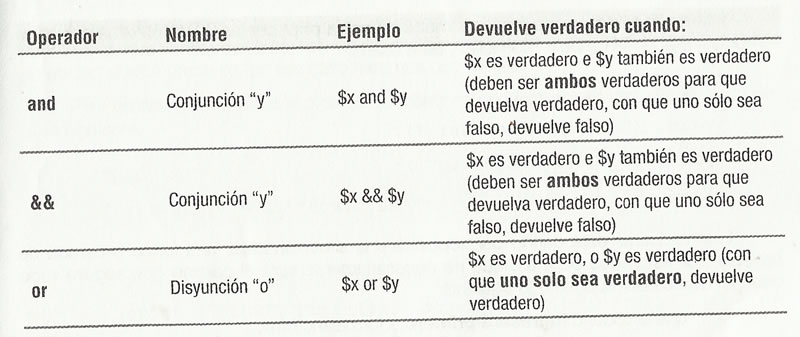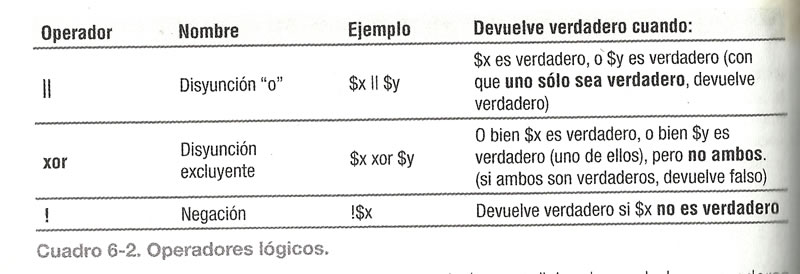Having understood the operation of conditional and comparison and logical operators, we are ready to perform different validations in our codes.

## Did you like it or was it useful?

Help us share it in Social Networks

Professor at the University of Guadalajara

Professional in Web Development and SEO Positioning for more than 10 continuous years.
We have more than 200 certificates and recognitions in the Academic and Professional trajectory, including diploma certificates certified by Google.

## IT ALSO DESERVES TO PAY TO VISIT:

### PREVIOUS

Logical operators in PHP

### NEXT

Validations in PHP